#### 期刊菜单

An Experimental Study on the Development of Chinese Tone Acquisition among Thai Students
DOI: 10.12677/ML.2023.111042, PDF, HTML, XML, 下载: 25  浏览: 73

Abstract: Relying on the research method of experimental phonetics and the Praat phonetic analysis software, this research compares and analyzes the current situation of Thai students’ acquisition of Chinese single and double tones at different stages. The Yinping tones of the students at the entry stage are generally low, and the students at the primary stage have improved. Thai students at all stages have a phenomenon of first falling and then rising in Yangping tones, showing a tendency of “fossilization”, and the degree of deviation is relatively serious; in the entry stage, they have not mastered the correct pronunciation of Shangsheng at all. In the primary stage, the tone value and tone type have been improved, but it is still not accurate enough. In addition, in the pronunciation of double-character tones, there are common problems in all stages that only drop but not rise or the starting value of the tone is too high. In the entry-level stage, the tone-removing is mainly manifested as a bias such as the inability to lower the tone, and there is basically no bias problem in the primary stage.

1. 引言

2. 实验设计

2.1. 实验发音人及录音Table 1. Speaker information form

2.2. 实验语料

2.3. 实验数据的提取和处理

2.3.1. 基频的提取和处理

2.3.2. 基频数据的统计与画图

2.3.3. 数据归一化处理

3. 实验结果与分析

3.1. 泰国学生单字调习得发展实验分析

3.1.1. 阴平调实验结果Table 4. Table of T values for Thai students’ Yinping single-syllable tone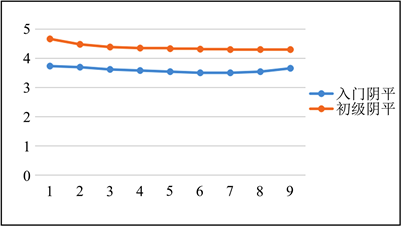Figure 1. Line chart of Thai students’ Yinping single-character tone acquisition

3.1.2. 阳平调实验结果Table 5. Table of T values for Thai students’ Yangping single-syllable tone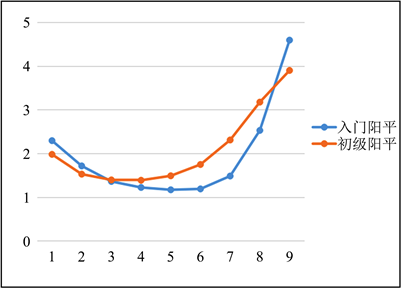Figure 2. Line chart of Thai students Yangping single-character tone acquisition

3.1.3. 上声调实验结果Table 6. Table of T values for Thai students’ Shangsheng single-syllable tone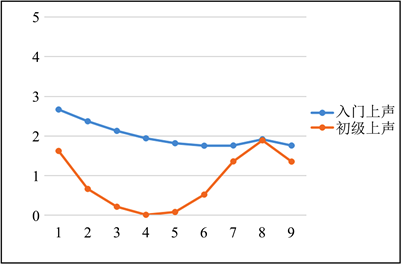Figure 3. Line chart of Thai students Shangsheng single-character tone acquisition

3.1.4. 去声调实验结果Table 7. Table of T values for Thai students’ Qusheng single-syllable tone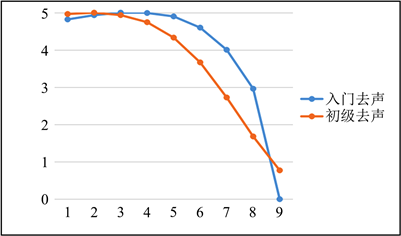Figure 4. Line chart of Thai students Qusheng single-character tone acquisition

3.2. 泰国学生双字调习得发展实验分析

3.2.1. “阴平 + X”声调组合Table 8. Table of T value of “Yinping + Yinping” two-character tones for Thai students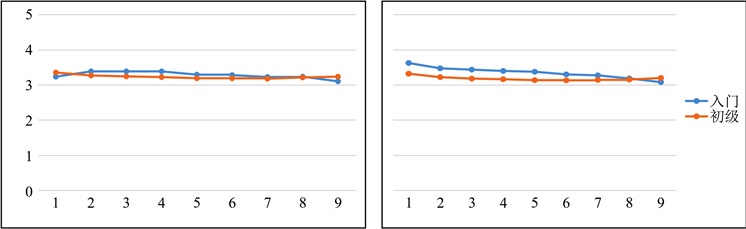Figure 5. Line chart of Thai students’ tone combination of “Yinping + Yinping”Table 9. Table of T value of “Yinping + Yangping” two-character tones for Thai students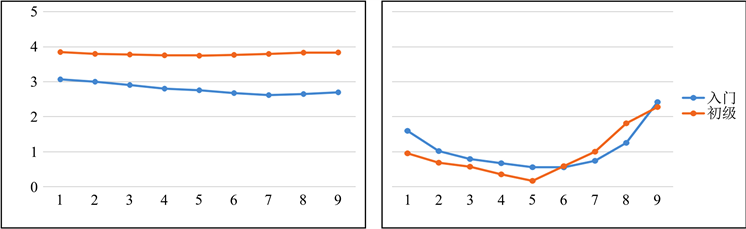Figure 6. Line chart of Thai students’ tone combination of “Yinping + Yangping”Table 10. Table of T value of “Yinping + Shangsheng” two-character tones for Thai students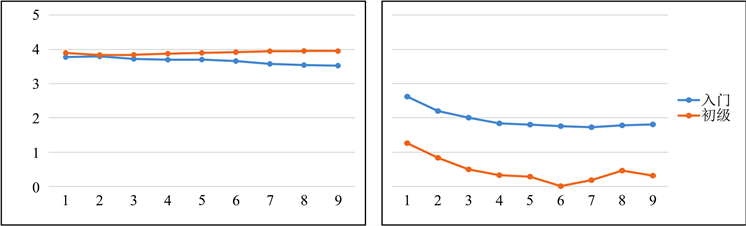Figure 7. Line chart of Thai students’ tone combination of “Yinping + Shangsheng”Table 11. Table of T value of “Yinping + Qusheng” two-character tones for Thai students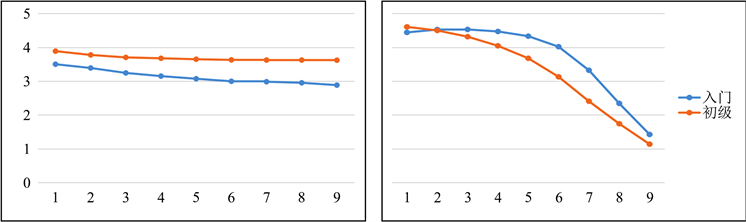Figure 8. Line chart of Thai students’ tone combination of “Yinping + Qusheng”

3.2.2. “阳平 + X”声调组合Table 12. Table of T value of “Yangping + Yinping” two-character tones for Thai students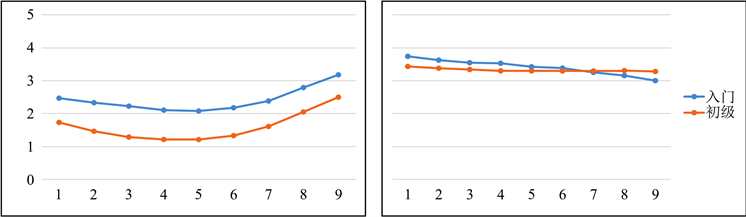Figure 9. Line chart of Thai students’ tone combination of “Yangping + Yinping”Table 13. Table of T value of “Yangping + Yangping” two-character tones for Thai students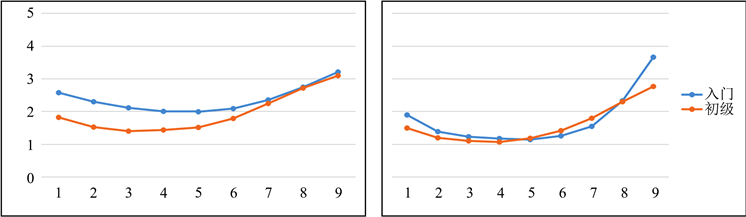Figure 10. Line chart of Thai students’ tone combination of “Yangping + Yangping”Table 14. Table of T value of “Yangping + Shangsheng” two-character tones for Thai students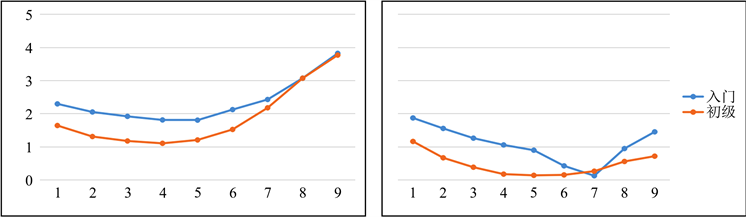Figure 11. Line chart of Thai students’ tone combination of “Yangping + Shangsheng”Table 15. Table of T value of “Yangping + Qusheng” two-character tones for Thai students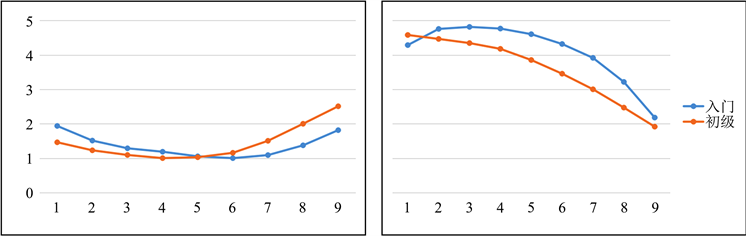Figure 12. Line chart of Thai students’ tone combination of “Yangping + Qusheng”

3.2.3. “上声 + X”声调组合Table 16. Table of T value of “Shangsheng + Yinping” two-character tones for Thai students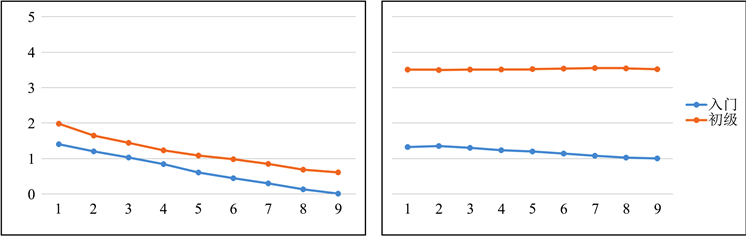Figure 13. Line chart of Thai students’ tone combination of “Shangsheng + Yinping”Table 17. Table of T value of “Shangsheng + Yangping” two-character tones for Thai students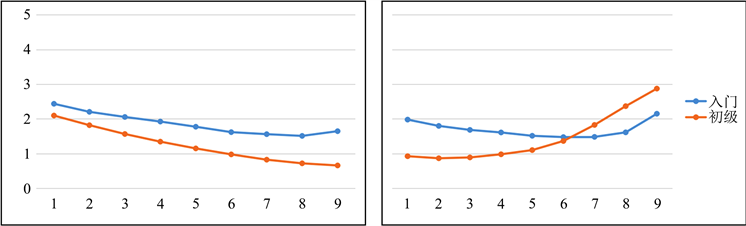Figure 14. Line chart of Thai students’ tone combination of “Shangsheng + Yangping”Table 18. Table of T value of “Shangsheng + Qusheng” two-character tones for Thai students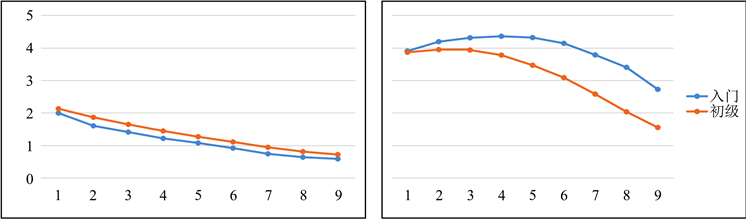Figure 15. Line chart of Thai students’ tone combination of “Shangsheng + Qusheng”

3.2.4. “去声 + X”声调组合Table 19. Table of T value of “Qusheng + Yinping” two-character tones for Thai students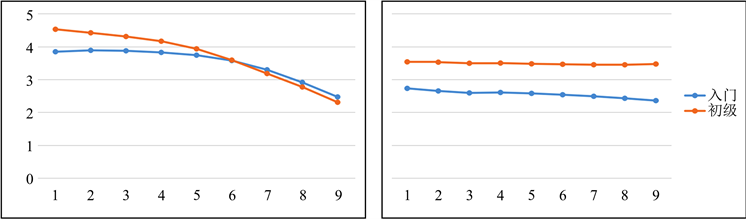Figure 16. Line chart of Thai students’ tone combination of “Qusheng + Yinping”Table 20. Table of T value of “Qusheng + Yangping” two-character tones for Thai students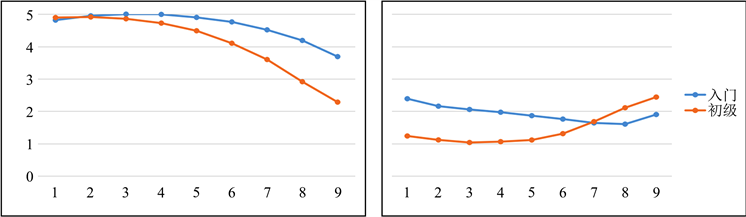Figure 17. Line chart of Thai students’ tone combination of “Qusheng + Yangping”Table 21. Table of T value of “Qusheng + Shangsheng” two-character tones for Thai students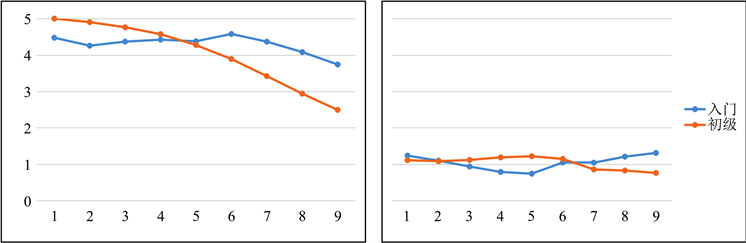Figure 18. Line chart of Thai students’ tone combination of “Qusheng + Shangsheng”Table 22. Table of T value of “Qusheng + Qusheng” two-character tones for Thai students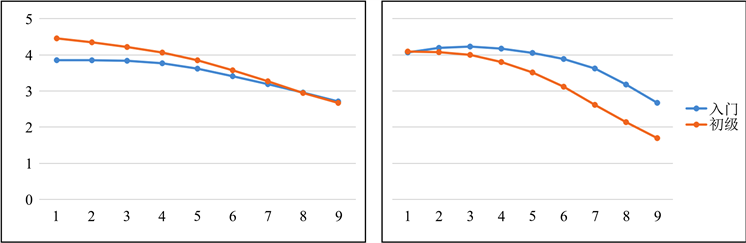Figure 19. Line chart of Thai students’ tone combination of “Qusheng + Qusheng”

3.3. 入门及初级阶段泰国学习者双字调习得偏误小结

4. 对泰声调教学对策与建议

4.1. 强化调值、调域、调型意识，重视双字调教学

4.2. 利用现代化技术手段，借助语音分析软件，进行对比分析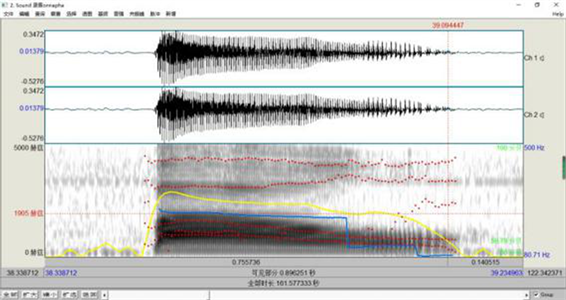Figure 20. Schematic diagram of the pronunciation of the word “full” for Thai students

4.3. 合理选择教材，改善课堂教学

  李红印. 泰国学生汉语学习的语音偏误[J]. 世界汉语教学, 1995(2): 66-71.  石锋. 实验音系学与汉语语音分析[J]. 南开语言学刊, 2006(2): 10-25+164.  贝先明, 向柠. 实验语音学的基本原理与praat软件操作[M]. 长沙: 湖南师范大学出版社, 2016: 10-35.  石锋. 语音学探微[M]. 北京: 北京大学出版社, 1990: 20-35.  薛晶晶. 美国和泰国学习者汉语普通话阳平与上声习得的实验研究[D]: [硕士学位论文]. 北京: 北京大学, 2013.  林茂灿. 汉语语调与声调[J]. 语言文字应用, 2004(3): 57-67.  郑琪. 中级汉语水平中亚留学生双字调声调偏误研究[J]. 语言与翻译, 2020(2): 87-90.  康小明, 武和平. 东干族留学生汉语单字调感知及产出声学实验研究[J]. 海外华文教育, 2020(1): 58-68.